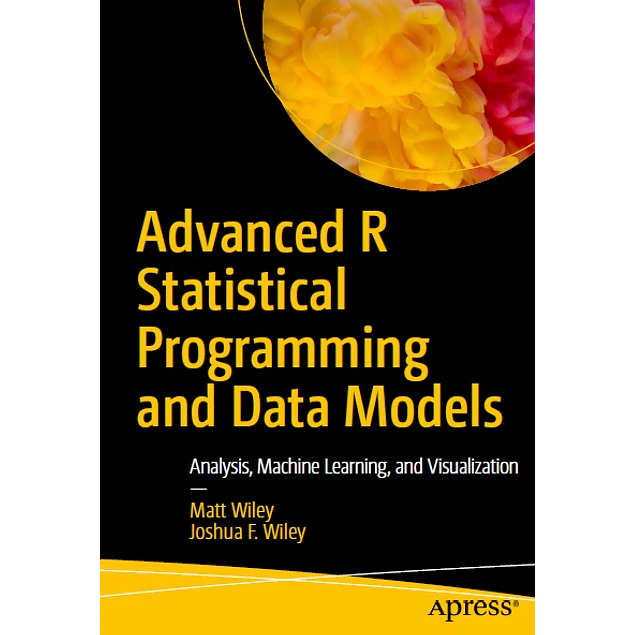## Advanced R Statistical Programming and Data Models: Analysis, Machine Learning, and Visualization

10.00\$# Advanced R Statistical Programming and Data Models: Analysis, Machine Learning, and Visualization

10.00\$

1st ed. 2019 Edition

by Matt Wiley (Author), Joshua F. Wiley (Author)

Carry out a variety of advanced statistical analyses including generalized additive models, mixed effects models, multiple imputation, machine learning, and missing data techniques using R. Each chapter starts with conceptual background information about the techniques, includes multiple examples using R to achieve results, and concludes with a case study.

Written by Matt and Joshua F. Wiley, Advanced R Statistical Programming and Data Models shows you how to conduct data analysis using the popular R language. You’ll delve into the preconditions or hypothesis for various statistical tests and techniques and work through concrete examples using R for a variety of these next-level analytics.  This is a must-have guide and reference on using and programming with the R language.

What You’ll Learn

• Conduct advanced analyses in R including: generalized linear models, generalized additive models, mixed effects models, machine learning, and parallel processing
• Carry out regression modeling using R data visualization, linear and advanced regression, additive models, survival / time to event analysis
• Handle machine learning using R including parallel processing, dimension reduction, and feature selection and classification
• Address missing data using multiple imputation in R
• Work on factor analysis, generalized linear mixed models, and modeling intraindividual variability

Who This Book Is For
Working professionals, researchers, or students who are familiar with R and basic statistical techniques such as linear regression and who want to learn how to use R to perform more advanced analytics. Particularly, researchers and data analysts in the social sciences may benefit from these techniques. Additionally, analysts who need parallel processing to speed up analytics are given proven code to reduce time to result(s).

Year:
2019
Pages:
649
Language:
English
Format:
PDF
Size:
70 MB
ISBN-10:
1484228715
ISBN-13:
978-1484228715
ASIN:
B07P2CTCM3
##### Contact
•••••